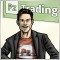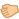# Lowest/Highest of closing prices in a given range (?)797

Good afternoon,

I have quite a weird problem, I am trying to extract the highest and lowest of all closing prices of a given period without sucess. This is what I have so far.

```double DCloseHighest(int shift, int period)
{
return(iHigh(Symbol(),Period(),iHighest(Symbol(), Period(), MODE_CLOSE, period+1,shift+1)));
}

double DCloseLowest(int shift, int period)
{
return(iLow(Symbol(),Period(),iLowest(Symbol(), Period(), MODE_CLOSE, period+1,shift+1)));
}```

However, the absolute high and low are returned, respectively. What am I doing wrong? :-)17205

flaab:

Good afternoon,

I have quite a weird problem, I am trying to extract the highest and lowest of all closing prices of a given period without sucess. This is what I have so far.

However, the absolute high and low are returned, respectively. What am I doing wrong? :-)

Thats what you are asking for . . .

```return( iHigh
return( iLow```797

RaptorUK:

Thats what you are asking for . . .

My bad. Stupid mistake. Got it. Thanks!

```double DCloseHighest(int shift, int period)
{
return(iClose(Symbol(),Period(),iHighest(Symbol(), Period(), MODE_HIGH, period+1,shift+1)));
}

double DCloseLowest(int shift, int period)
{
return(iClose(Symbol(),Period(),iLowest(Symbol(), Period(), MODE_LOW, period+1,shift+1)));
}```17205

flaab:

My bad. Stupid mistake. Got it. Thanks!

Easily done,  you are welcome369

flaab:

My bad. Stupid mistake. Got it. Thanks!

```double DCloseHighest(int shift, int period)
{
return(iClose(Symbol(),Period(),iHighest(Symbol(), Period(), MODE_HIGH, period+1,shift+1)));
}

double DCloseLowest(int shift, int period)
{
return(iClose(Symbol(),Period(),iLowest(Symbol(), Period(), MODE_LOW, period+1,shift+1)));
}```

If you are looking for the highest/lowest close price, I believe you need to use MODE_CLOSE in your iHighest() function.

```double DCloseHighest(int shift, int period)
{
return(iClose(Symbol(),Period(),iHighest(Symbol(), Period(), MODE_CLOSE, period+1,shift+1)));
}

double DCloseLowest(int shift, int period)
{
return(iClose(Symbol(),Period(),iLowest(Symbol(), Period(), MODE_CLOSE, period+1,shift+1)));
}```797

Thirteen:

If you are looking for the highest/lowest close price, I believe you need to use MODE_CLOSE in your iHighest() function.

As weird as it might be, it returns exactly the same data.17205

flaab:
As weird as it might be, it returns exactly the same data.
Coincidence I reckon . . .   use MODE_CLOSE